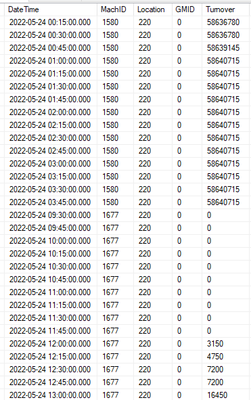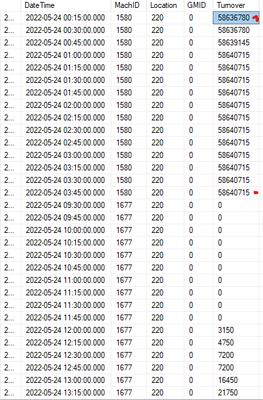cancel
Showing results for
Did you mean:Frequent Visitor

## How to choose the earliest value of the column and finish the calculation?

Hi, please give me some help.

The column's values are continuous but they were broken by 0.

I need to use the maximum value(58640715) to subtract the earliest value(58636780). Max(turnover)-earliest(value)

Could you please tell me how to filter the earliest value(58636780)?

The columns likes this.Thanks,

1 ACCEPTED SOLUTIONCommunity Support

Hi @Bridge ,

Are you trying to calculate the max value and min(except 0) value for the same match_ID?

If so you could try this formula:

``````column =
VAR _max =
CALCULATE (
MAX ( 'table'[turnover] ),
ALLEXCEPT ( 'table', 'table'[match_id] )
)
VAR _min =
CALCULATE (
MIN ( 'table'[turnover] ),
FILTER ( ALLEXCEPT ( 'table', 'table'[match_id] ), 'table'[turnover] <> 0 )
)
RETURN
_max - _min
``````

Best Regards,

Jay

Community Support Team _ Jay
If this post helps, then please consider Accept it as the solution
to help the other members find it.
5 REPLIES 5Community Support

Hi @Bridge ,

Are you trying to calculate the max value and min(except 0) value for the same match_ID?

If so you could try this formula:

``````column =
VAR _max =
CALCULATE (
MAX ( 'table'[turnover] ),
ALLEXCEPT ( 'table', 'table'[match_id] )
)
VAR _min =
CALCULATE (
MIN ( 'table'[turnover] ),
FILTER ( ALLEXCEPT ( 'table', 'table'[match_id] ), 'table'[turnover] <> 0 )
)
RETURN
_max - _min
``````

Best Regards,

Jay

Community Support Team _ Jay
If this post helps, then please consider Accept it as the solution
to help the other members find it.Super User

Hi,

Show the exact result you are expecting.  Also, share data in a format that one can work on

Regards,
Ashish Mathur
http://www.ashishmathur.comFrequent Visitor

Sorry for my unclear description.

I want to get the result of the maximum value - the earliest value

The Earliest value(58636780) happened on 2022-05-24 00:15:00.000. It's the earliest value that happened on the day.

I use the Max function to find the max value(58640715). But I don't know how to write the function to find the earliest value.Super User

Hi,

Do you want this as a calculated column formula or as a measure in the visual.  Share the download link of your PBI file.

Regards,
Ashish Mathur
http://www.ashishmathur.comFrequent Visitor

I ever used the formula like

MINX(Filter(Table, MIN(DateTime), Turnover),

But it didn't work.